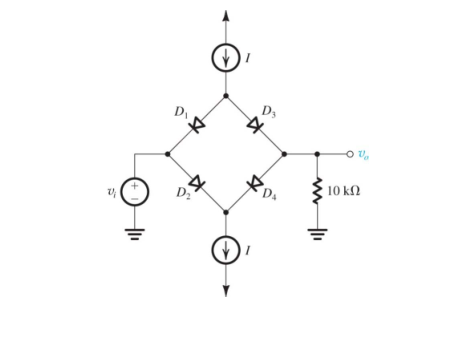Create an Account

Home / Questions / In the circuit shown in the figure below diodes D 1 through D 4 are identical and each exh...

In the circuit shown in the figure below diodes D 1 through D 4 are identical and each exhibits a voltage drop of 0 7 V at a 1 mA current

In the circuit shown in the figure below, diodes D 1 through D 4 are identical, and each exhibits a
voltage drop of 0.7 V at a 1-mA current.
(a) For small input signals (e.g., 10-mV peak), find the small-signal equivalent circuit and use
it to determine values of the small-signal transmission v o /v i for various values of I: 0 A????1A, 1
A????1A, 10 A????1A, 100 A????1A, 1 mA, and 10 mA.
(b) For a forward-conducting diode, what is the largest signal-voltage magnitude that it can
support while the corresponding signal current is limited to 10% of the dc bias current?
Now, for the circuit in the figure below, for 10-mV peak input, what is the smallest value
of I for which the diode currents remain within A????110% of their dc values?
(c) For I = 1 mA, what is the largest possible output signal for which the diode currents deviate
by at most 10% of their dc values? What is the corresponding peak input? What is the total
current in each diode?Show transcribed image text In the circuit shown in the figure below, diodes D 1 through D 4 are identical, and each exhibits a voltage drop of 0.7 V at a 1-mA current. (a) For small input signals (e.g., 10-mV peak), find the small-signal equivalent circuit and use it to determine values of the small-signal transmission v o /v i for various values of I: 0 A, 1 A, 10 A, 100 A, 1 mA, and 10 mA. (b) For a forward-conducting diode, what is the largest signal-voltage magnitude that it can support while the corresponding signal current is limited to 10% of the dc bias current? Now, for the circuit in the figure below, for 10-mV peak input, what is the smallest value of I for which the diode currents remain within 10% of their dc values? (c) For I = 1 mA, what is the largest possible output signal for which the diode currents deviate by at most 10% of their dc values? What is the corresponding peak input? What is the total current in each diode?

Jul 31 2020 View more View LessSubscribe To Get Solution